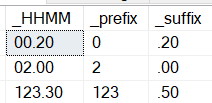# HH:MM Accumulative Time format Conversion from Decimal

Hi,

I have this

``````CREATE function [dbo].[sp_DecToHHMM]
(@time DECIMAL(5,2))
RETURNS nvarchar(30)
As
BEGIN

select @additional=replace(cast(convert(decimal(10,2),cast(@time as int)+((@time-cast(@time as int))*.60)) as varchar),'.',':')
END
``````

When I run this:-

select [dbo].[sp_DecToHHMM] ( 2 ) output: 2:00 I want 02:00
select [dbo].[sp_DecToHHMM] ( 123.50 ) output 123:30

Regards,
Micheale

``````ALTER function [dbo].[sp_DecToHHMM]
(@time DECIMAL(5,2))
RETURNS nvarchar(30)
As
BEGIN

select @additional=replace(cast(convert(decimal(10,2),cast(@time as int)+((@time-cast(@time as int))*.60)) as varchar),'.',':')
END``````

Hi HarisHgg,

select [tnawebusr].[sp_DecToHHMM] ( 123.5 ) >> 0123:30
select [tnawebusr].[sp_DecToHHMM] ( 3.5 ) >> 03:30

Cannot work

explain
the different Data Input scenarios

and how you would like the OUTPUT ..

Hi,

select [dbo].[sp_DecToHHMM] ( 2 ) output desire: 02:00 >> NOT work - Now showing 2:00
select [dbo].[sp_DecToHHMM] ( 123.50 ) output desire:123:30 >> Work
select [dbo].[sp_DecToHHMM] ( 0.2 ) output desire: 00:12 >> NOT Work - Now showing 0:12
select [dbo].[sp_DecToHHMM] ( 0.2 )

I want if the before : length is 1, then add 0 infront else return same value without adding '0'+ infront

Thanks.

Regards,
Micheale

Cast as integer

Example data
1.789

1

Check the length using len function

If length equals 1 append 0

Otherwise don't do anything

Declare @var int
Select @var = cast ( your date time ) as int

If len(@var) = 1
Return '0' + returndata

What is the purpose of this function? Is it for report display purposes?

You are conflating too many disparate data types and expecting a result that is not what the function name indicates
sp_DecToHHMM but some output it
HHH:MM

02:00 is not really HH:MM because there is no 02 hours that I know of unless its a time format where you are. Is that pm or am? So again what is end game of this function what is it being used for?

Hi,

Actually is a total working hour. I have a decimal of work hour, I want convert it to be in Time Format.

Decimal =>Hours. Example that I want to achieve:-
0.2 = > 00:12
2 => 02:00
123.50 => 123:30

Thanks

Regards,
Micheale

Please list out all the possible values after the fraction
50 is 30 what other values could show up?

hi micnie

this is how far i got

the issue of getting 2 decimal part is there ..

hope this helps

``````CREATE function [dbo].[sp_DecToHHMM]
(@time DECIMAL(5,2))
RETURNS
nvarchar(30)
As
BEGIN

declare @return_int varchar(100) = ''

if len(PARSENAME(@Time,2)) = 1
set @return_int =  '0'+(PARSENAME(@Time,2))+':'+cast(PARSENAME(@Time,1)*60/100  as varchar(10))
else
set @return_int = (PARSENAME(@Time,2))+':'+cast(PARSENAME(@Time,1)*60/100  as varchar(10))

return @return_int
END
go
``````

I would recommend you do not use a function, do it inline

``````
declare @foofoo table(formaggio decimal(5,2))

insert into @foofoo
select 0.2 union
select 2 union
select 123.50

;with src
as
(
select substring(cast(formaggio as varchar(100)),
0,charindex('.',formaggio)) as _prefix,
substring(cast(formaggio as varchar(100)),
charindex('.',formaggio), len(formaggio)) as _suffix

from @foofoo src
)
select case
when len(_prefix) = 1 then '0' + _prefix
else _prefix
end + case when _suffix = '.50' then '.30'
else _suffix
end as _HHMM
from src
``````also as a variable

``````declare @notreaalytime decimal(5,2) = 0.2

;with src
as
(
select substring(cast(@notreaalytime as varchar(100)), 0,charindex('.',@notreaalytime)) as _prefix,
substring(cast(@notreaalytime as varchar(100)),charindex('.',@notreaalytime), len(@notreaalytime)) as _suffix

--from @foofoo src
)
select case
when len(_prefix) = 1 then '0' + _prefix
else _prefix
end + case when _suffix = '.50' then '.30'
else _suffix
end as _HHMM,
_prefix,
_suffix
from src
``````
``````ALTER  FUNCTION [dbo].[sp_DecToHHMM]
(@time DECIMAL(5,2))
RETURNS nvarchar(30)
As
BEGIN
RETURN (
SELECT CASE WHEN LEN(FLOOR(@time)) = 1 THEN '0' ELSE '' END +
CAST(FLOOR(@time) AS nvarchar(20)) + N':' +
RIGHT('0' + CAST(FLOOR((@time - FLOOR(@time)) * 60) AS nvarchar(2)), 2) AS hhmm
)
END``````

Thanks everyone.